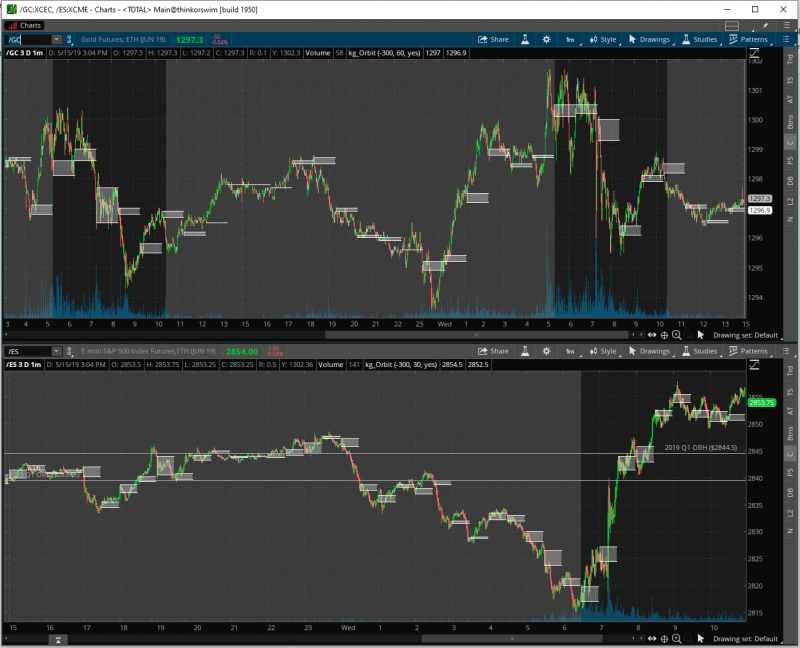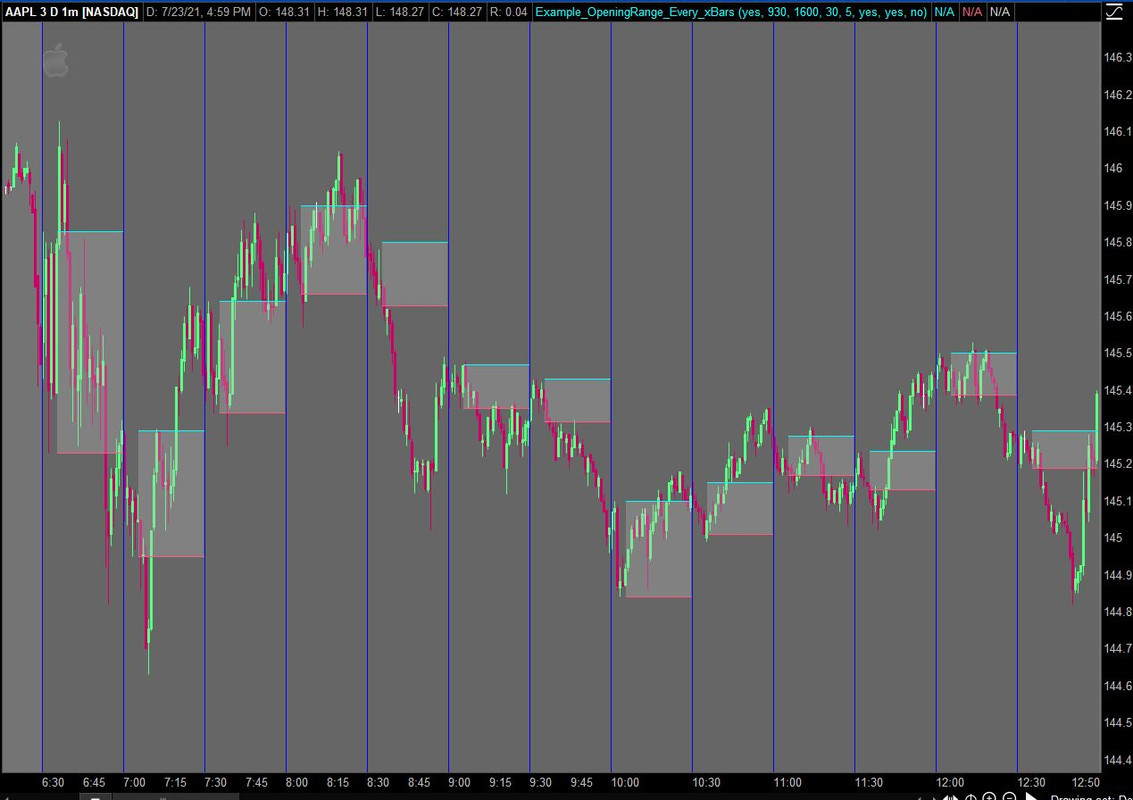# Opening Range every N Minutes For ThinkOrSwim

#### korygill

##### Well-known member
VIP
This study displays an Opening Range (OR) on a chart every N minutes. It can be configured to display only during trading hours, or all hours. The code has only been tested on 3-day 1-minute charts for 30 and 60 minute OR bars. The full usefulness of this study is unknown and is left to the user. There are many times during the day where an Opening Range can provide insight into market action, and perhaps this will help users discover these.thinkScript Code

Rich (BB code):
``````# Orbit
#
# Description
# Draws the Opening Range (OR) for each recurring time period.
# Suggested use on 3d/1m chart with 30 min or 60 min ranges.
#
# Author: Kory Gill, @korygill
#
# Comment out unnecessary portions to preserve TOS memory and enhance speed
#

input timeOffsetFromEST = -300; #hint timeOffsetFromEST: 24-hour time offset from EST (-300 for PST)

input frequencyInMinutes = 60; #hint frequencyInMinutes: 30 mins or 60 mins recommended

input showAllMarketHours = no; #hint showAllMarketHours: if yes, all hours highlighted, not just market hours

# Common variables. Using variables reduces calls to TOS iData server.

# iData Definitions
def vHigh = high;
#def initHigh =  CompoundValue(1, high, high);  # creates an initialized variable for high
def vLow = low;
#def initLow = CompoundValue(1, low, low);
#def vOpen = open;
#def initOpen = CompoundValue(1, open, open);
#def vClose = close;
#def initClose = CompoundValue(1, close, close);
#def vVolume = volume;
#def initVolume = CompoundValue(1, volume, volume);
def nan = Double.NaN;

# Bar Time & Date
#def bn = BarNumber();
#def currentBar = HighestAll(if !IsNaN(vHigh) then bn else nan);
#def Today = GetDay() ==GetLastDay();
#def time = GetTime();
#def GlobeX = GetTime() < RegularTradingStart(GetYYYYMMDD());
#def globeX_v2 = if time crosses below RegularTradingEnd(GetYYYYMMDD()) then bn else GlobeX;
#def RTH = GetTime() > RegularTradingStart(GetYYYYMMDD());
#def RTH_v2 = if time crosses above RegularTradingStart(GetYYYYMMDD()) then bn else RTH;

# Bars that start and end the sessions
#def rthStartBar    = CompoundValue(1,
#                         if   !IsNaN(vClose)
#                         &&   time crosses above RegularTradingStart(GetYYYYMMDD())
#                         then bn
#                         else rthStartBar, 0);
#def rthEndBar      = CompoundValue(1,
#                         if   !IsNaN(vClose)
#                         &&   time crosses above RegularTradingEnd(GetYYYYMMDD())
#                         then bn
#                         else rthEndBar, 1);
#def globexStartBar = CompoundValue(1,
#                         if   !IsNaN(vClose)
#                         &&   time crosses below RegularTradingEnd(GetYYYYMMDD())
#                         then bn
#                         else globexStartBar, 1);
#def rthSession = if bn crosses above rthStartBar #+ barsExtendedBeyondSession
#                    then 1
#                    else if   bn crosses above rthEndBar #+ barsExtendedBeyondSession
#                         then 0
#                    else rthSession;

#
# Settings for well-known symbols
#
def startTime;

if (GetSymbol() == "/ES:XCME" or GetSymbol() == "/MES:XCME") {
startTime = 0630 - timeOffsetFromEST;
}
else if (GetSymbol() == "/NQ:XCME" or GetSymbol() == "/MNQ:XCME") {
startTime = 0630 - timeOffsetFromEST;
}
else if (GetSymbol() == "/YM:XCBT" or GetSymbol() == "/MYM:XCBT") {
startTime = 0630 - timeOffsetFromEST;
}
else if (GetSymbol() == "/RTY:XCME" or GetSymbol() == "/M2K:XCME") {
startTime = 0630 - timeOffsetFromEST;
}
else if (GetSymbol() == "/CL:XNYM") {
startTime = 0600 - timeOffsetFromEST;
}
else if (GetSymbol() == "/QM:XNYM") {
startTime = 0600 - timeOffsetFromEST;
}
else if (GetSymbol() == "/GC:XCEC") {
startTime = 0520 - timeOffsetFromEST;
}
else if (GetSymbol() == "/SI:XCEC") {
startTime = 0525 - timeOffsetFromEST;
}
else if (GetSymbol() == "/6J:XCME") {
startTime = 0520 - timeOffsetFromEST;
# there is a bug somewhere using 1200 I saw once.
# if you have issues, use 1159 below.
}
else {
# have to have an else...guess
startTime = 0630 - timeOffsetFromEST;
}

def modMinutes = (SecondsFromTime(startTime) % (frequencyInMinutes*60)) / 60;
#plot cm = modMinutes;

def showBar =  modMinutes != (frequencyInMinutes - 1) and  modMinutes != -1;
#plot sb = showBar;

def orbitActive = if showAllMarketHours or
SecondsFromTime(startTime) >= 0)
then 1
else 0;

def orbitHigh = if orbitActive and modMinutes == 0
then vHigh
else orbitHigh;

def orbitLow = if orbitActive and modMinutes == 0
then vLow
else orbitLow;

plot pOrbitHigh = if orbitActive and showBar and orbitHigh != 0 then orbitHigh else nan;
plot pOrbitLow = if orbitActive and showBar and orbitLow != 0 then orbitLow else nan;

pOrbitHigh.SetDefaultColor(Color.WHITE);
pOrbitLow.SetDefaultColor(Color.WHITE);

#def orbitWidth = orbitHigh - orbitLow;
#plot pOrbitWidth = if orbitActive and showBar and orbitWidth != 0 then orbitWidth else nan;
#pOrbitWidth.Hide();

https://tos.mx/TKC4oP

#### Attachments

Last edited:
•Txsroper and skynetgen

#### futures252

##### New member
Hi, I've been trying to modify your script to redraw the lines whenever price passes either the high or the low instead of having it redraw every n minutes. I wasn't able to do so, wondering if you can help?

thanks

#### SimpleStock

##### New member
Would it be possible to change this script to display first 5 minute range every half hour on a 1 minute chart?

#### SleepyZ

##### Well-known member
VIP
Would it be possible to change this script to display first 5 minute range every half hour on a 1 minute chart?

See if this is what you want. You can change the interval at input minutes and the range at input range.Ruby:
``````input show_buy_sell_bubbles = yes;
input begin = 0930;
input end   = 1600;
def bar = if SecondsFromTime(begin) < 0 and SecondsTillTime(end) > 0
then 0
else if SecondsTillTime(begin) == 0 and
SecondsFromTime(begin) == 0
then 0
else bar + 1;
input minutes = 30;
def barx_min  = bar % ((minutes) / (GetAggregationPeriod() / 60000)) == 0;
def barxct    = if barx_min then 1 else barxct + 1;

input range  = 5;
def today    = SecondsFromTime(begin) >= 0 and SecondsFromTime(end) <= 0;
def ORActive = if SecondsFromTime(begin) < 0 and SecondsTillTime(end) > 0 then 0 else if Between(barxct, 1, range) then 1 else 0;

rec ORHigh = if ORActive == 0 and  ORActive == 1 then high else if ORActive and high > ORHigh then high else ORHigh;
rec ORLow  = if ORActive == 0 and ORActive == 1 then low else if ORActive and low < ORLow then low else ORLow;

plot hrange = if !today or ORActive then Double.NaN else ORHigh;
plot lrange = if !today or ORActive then Double.NaN else ORLow;

input show_cloud = yes;
AddCloud(if show_cloud then hrange else Double.NaN, lrange, Color.LIGHT_GRAY);

input showverticalline = yes;
AddVerticalLine(showverticalline and today and barx_min, "", Color.BLUE, stroke = Curve.FIRM);

input debug = no;
plot x = if debug then ORActive else Double.NaN ;
x.SetPaintingStrategy(PaintingStrategy.VALUES_BELOW);``````

•SimpleStock

#### SimpleStock

##### New member
See if this is what you want. You can change the interval at input minutes and the range at input range.
that's it.... thank you.
At the same time... is it possible to extend the previous range during ORActive? I added breakout indicator but it would be accurate when previous range is plotted during ORActive. I ultimately love to create a breakout system based on this indicator, at least for testing and see results.

Sorry... Don't know how to add image to this but I had marked it on one chart.

Last edited:

#### SleepyZ

##### Well-known member
VIP
that's it.... thank you.
At the same time... is it possible to extend the previous range during ORActive? I added breakout indicator but it would be accurate when previous range is plotted during ORActive. I ultimately love to create a breakout system based on this indicator, at least for testing and see results.

Sorry... Don't know how to add image to this but I had marked it on one chart.

Just noticed your request for extended lines from the previous range.

Ruby:
``````input show_buy_sell_bubbles = yes;
input begin = 0930;
input end   = 1600;
def bar = if SecondsFromTime(begin) < 0 and SecondsTillTime(end) > 0
then 0
else if SecondsTillTime(begin) == 0 and
SecondsFromTime(begin) == 0
then 0
else bar + 1;
input minutes = 30;
def barx_min  = bar % ((minutes) / (GetAggregationPeriod() / 60000)) == 0;
def barxct    = if barx_min then 1 else barxct + 1;

input range  = 5;
def today    = SecondsFromTime(begin) >= 0 and SecondsFromTime(end) <= 0;
def ORActive = if SecondsFromTime(begin) < 0 and SecondsTillTime(end) > 0 then 0 else if Between(barxct, 1, range) then 1 else 0;

rec ORHigh = if ORActive == 0 and  ORActive == 1 then high else if ORActive and high > ORHigh then high else ORHigh;
rec ORLow  = if ORActive == 0 and ORActive == 1 then low else if ORActive and low < ORLow then low else ORLow;

plot hrange = if !today or ORActive then Double.NaN else ORHigh;
plot lrange = if !today or ORActive then Double.NaN else ORLow;

def orhigh1  = if barxct == 1 then hrange else orhigh1;
def orlow1   = if barxct == 1 then lrange else orlow1;

plot hrange1 = orhigh1;
plot lrange1 = orlow1;

hrange1.SetPaintingStrategy(PaintingStrategy.HORIZONTAL);
lrange1.SetPaintingStrategy(PaintingStrategy.HORIZONTAL);
hrange1.SetDefaultColor(Color.CYAN);
lrange1.SetDefaultColor(Color.YELLOW);

input show_cloud = yes;
AddCloud(if show_cloud then hrange else Double.NaN, lrange, Color.LIGHT_GRAY);

input showverticalline = yes;
AddVerticalLine(showverticalline and today and barx_min, "", Color.BLUE, stroke = Curve.FIRM);

input debug = no;
plot x = if debug then ORActive else Double.NaN ;
x.SetPaintingStrategy(PaintingStrategy.VALUES_BELOW);``````

### Not the exact question you're looking for?

87k+ Posts
229 Online## The Market Trading Game Changer

Join 2,500+ subscribers inside the useThinkScript VIP Membership Club
• Exclusive indicators
• Proven strategies & setups
• Private Discord community
• Exclusive members-only content
• 1 full year of unlimited support

What is useThinkScript?

useThinkScript is the #1 community of stock market investors using indicators and other tools to power their trading strategies. Traders of all skill levels use our forums to learn about scripting and indicators, help each other, and discover new ways to gain an edge in the markets.

How do I get started?

We get it. Our forum can be intimidating, if not overwhelming. With thousands of topics, tens of thousands of posts, our community has created an incredibly deep knowledge base for stock traders. No one can ever exhaust every resource provided on our site.

If you are new, or just looking for guidance, here are some helpful links to get you started.

What are the benefits of VIP Membership?